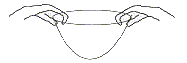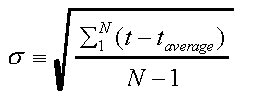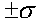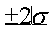# Experiment of The Month

## Filter drop and statistics

The MU Physics Department does not claim that we invented these labs. Actually, the origin of these labs are currently unknown to us.

We use this lab to introduce the ideas of average and standard deviation. It can be extended to study the question of how things fall. (Do falling objects move with constant velocity? constant acceleration? some other way?)

The falling objects in this case are paper coffee filters. We use either cone filters (mashed with a fist into roughly the shape of a rocket nose cone) and basket filters (used as they are packaged). If the cone filters are not shaped right, they can sail in a non-reproducible fashion. The basket filters must be dropped using two hands to get a "straight" fall.Students work with partners. One student drops a filter from about 2 meters above the floor. A second student starts a stop watch when the filter is 1 meter above the floor, and stops the watch when the filter hits the floor. Each student takes 6 turns at dropping the filter and 6 turns at timing the 1 meter fall.

Each individual time is put on the black board in tabular form. A histogram of the times is formed from this table, and is discussed. It is proposed to represent the entire set of data with just 2 numbers: The average value of all of the times, and a number to represent the "width" of the distribution represented by the histogram. Average deviation and standard deviation are discussed, and we choose to use standard deviation,Pairs (or larger groups) combine their data and

1. plot a histogram
2. calculate the average and standard deviation
3. mark the average on the histogram
4. mark the intervalon the histogram
5. test the prediction that about 2/3 of the times will fall within one standard deviation of the average.
6. mark the intervalon the histogram
7. test the prediction that about 95% of the times fall within 2 standard deviations of the average.